# Robin problem

(diff) ← Older revision | Latest revision (diff) | Newer revision → (diff)

equilibrium problem, electrostatic problem

A problem on the distribution of a positive Borel measureon the boundaryof a compact setin the-dimensional Euclidean space,, which generates a constant Newton potential for, or constant logarithmic potential for, on any connected component of the interior of, i.e. the problem on the equilibrium distribution of an electric charge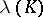on the surfaceof a conductor.

In the simplest classical case whenis a closed domain inhomeomorphic to the sphere, bounded by a smooth simple surface or (when) by a curveof class,,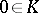, the solution of Robin's problem is reduced to finding a non-trivial solution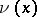,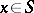, of the homogeneous Fredholm-type integral equation of the second kind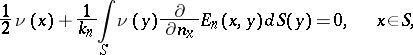(1)

under the normalization condition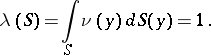(2)

Here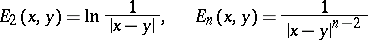for,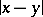is the distance between two points,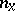is the direction of the exterior normal toat the point,is the derivative, or density, of the absolutely-continuous measurewith respect to the Lebesgue measure on,for, and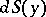is the area element of the surface. Equation (1) is obtained when one considers the interior Neumann problem for the domain bounded byunder vanishing boundary conditions, since the simple-layer potentialcalled the Robin potential, equilibrium potential or capacity potential, should, according to the condition of Robin's problem, have a constant value on(see Potential theory, and also ). The solutionfor the problem (1), (2) under the indicated conditions always exists in the class of continuous functions. The measure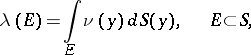which provides a solution of the Robin problem, is called the equilibrium measure. In a more complicated case, when the boundary of the compact setconsists of a finite number of non-intersecting simple closed surfaces or (when) curves of class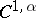,(see ), the Robin problem is solved in a similar way. Moreover, on bounded connected components of the open set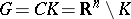the Robin potential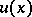also preserves its constant value, i.e. on the boundaries of these components the density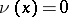.

Let the compact setbe connected. The constant value of the Robin potentialon,is called the Robin constant of the compact set. Forit is related to the harmonic, or Newton, capacity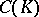ofby the simple relation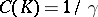; moreover,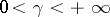,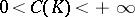. For, the Robin constant can assume all values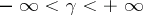; the harmonic capacity is then expressed by the formula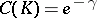.

In another way, the equilibrium measureis defined as the measure which yields the minimum of the energy integralin the class of all measuresconcentrated onand such that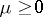,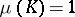. Such a measurein the case of a compact setwith a smooth boundary coincides with the one found above, but it exists also in the general case of an arbitrary compact set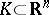,, if only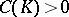. The corresponding equilibrium potentialwhich is a generalization of the Robin potential, preserves the constant valuefor, or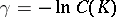for, everywhere onexcept perhaps at the points of some set of capacity zero.

The name "Robin problem" is connected with studies of G. Robin (see ).

How to Cite This Entry:
Robin problem. Encyclopedia of Mathematics. URL: http://encyclopediaofmath.org/index.php?title=Robin_problem&oldid=14200
This article was adapted from an original article by E.D. Solomentsev (originator), which appeared in Encyclopedia of Mathematics - ISBN 1402006098. See original article